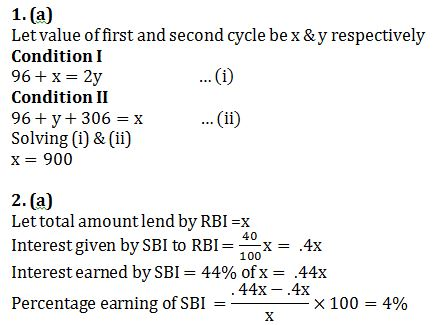# Quant Quiz 5th September 2018

Question1: Jonny has two cycles and one rickshaw. The rickshaw is worth Rs. 96. If he sells the rickshaw along with the first cycle, he has an amount double that of the value of the second cycle. But if he decides to sell the rickshaw along with the second cycle, the amount received would be less than the value of first cycle by Rs. 306. What is the value of first cycle?

(a) Rs. 900

(b) Rs. 600

(c) Rs. 498

(d) Rs. 520

(e) None of these

Question2: The RBI lends a certain amount to the SBI on simple interest for two years at 20%. The SBI gives this entire amount to Bharti Telecom on compound interest for two years at the same rate annually. Find the percentage earning of the SBI at the end of two years on the entire amount.

(a) 4%

(b) 3(1/7)%

(c) 3(2/7)%

(d) 3(6/7)%

(e) None of these

Question3: A mother divided an amount of Rs. 61,000 between her two daughters aged 18 years and 16 years respectively and deposited their shares in a bond. If the interest rate is 20% compounded annually and if each received the same amount as the other when they attained the age of 20 years, their shares are

(a) Rs. 35,600 and Rs. 25,400

(b) Rs. 30500 each

(c) Rs. 24,000 and Rs. 37000

(d) Rs. 35,000  and Rs 26,000

(e) None of these

Question4: There are three Taps A, B and C in a tank. They can fill the tank in 10 hrs, 20 hrs and 25 hrs respectively. At first, all of them are opened simultaneously. Then after 2 hours, tap C is closed and A and B are kept running. After the 4th hour, tap B is also closed. The remaining work is done by Tap A alone. Find the percentage of the work done by Tap A by itself.

(a) 32%

(b) 52%

(c) 75%

(d) 72%

(e) None of these

Question5: A boat sails downstream from point A to point B, which is 10 km away from A, and then returns to A. If the actual speed of the boat (in still water) is 3 km/h, the trip from A to B takes 8 hours less than that from B to A. What must the actual speed of the boat for the trip from A to B to take exactly 100 minutes?

(a) 1 km/h

(b) 2 km/h

(c) 3 km/h

(d) 4 km/h

(e) None of these

Directions (6-10): Study the following table carefully to answer the questions that follow.

The table given below provides the incomplete data related to monthly earning & expenditure of five friends. Find the missing value if required to answer the questions.

 Friends Total Income (In Rs) Ratio of Savings & Expenditure S : E Expenditure(in Rs) on Rent Food Others Soha – 5 : 6 20% 15% 7800 Ruchi 28000 5 : 9 – 16% 62% Suchi 22000 – : – 2000 2200 58% Meenu 26000 – : 6 15% 25% 7200 Teena – 8 : 9 4000 4000 10000

Question6: Find the annual income of soha.

(a) Rs 26,400

(b) Rs 2,65,000

(c) Rs 26,500

(d) Rs 2,64,000

(e) None of these

Question7: Find the difference in the monthly savings of Suchi and Meenu.

(a) Rs 1200

(b) Rs 2200

(c) Rs 2000

(d) Rs 1800

(e) None of these

Question8: Expenditure made by Ruchi on rent is what percent of expenditure made by Meenu on food?

(a) 32%

(b) 132%

(c) 88%

(d) 120%

(e) None of these

Question9: The savings of Ruchi is what percent more or less than that of Teena?

(a) 32%

(b) 37%

(c) 38%

(d) 40%

(e) None of these

Question10: Find the average of monthly income of Soha, Suchi and Teena.

(a) Rs 26500

(b) RS 26000

(c) Rs 25600

(d) Rs 22500

(e) None of these

## Solution